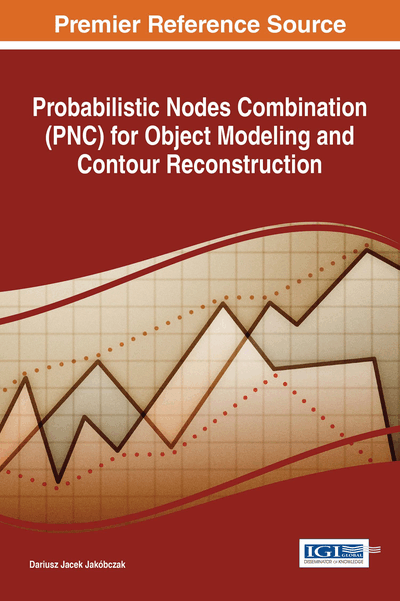# Probabilistic Nodes Combination (PNC): Formulas and Examples

DOI: 10.4018/978-1-5225-2531-8.ch002

## Abstract

The method of Probabilistic Nodes Combination (PNC) enables interpolation and modeling of two-dimensional curves using nodes combinations and different coefficients ?: polynomial, sinusoidal, cosinusoidal, tangent, cotangent, logarithmic, exponential, arc sin, arc cos, arc tan, arc cot or power function, also inverse functions. This probabilistic view is novel approach a problem of modeling and interpolation. Computer vision and pattern recognition are interested in appropriate methods of shape representation and curve modeling. PNC method represents the possibilities of shape reconstruction and curve interpolation via the choice of nodes combination and probability distribution function for interpolated points. It seems to be quite new look at the problem of contour representation and curve modeling in artificial intelligence and computer vision. Function for ? calculations is chosen individually at each curve modeling and it is treated as probability distribution function: ? depends on initial requirements and curve specifications.
Chapter Preview
Top

## Introduction

Probabilistic modeling is still a developing branch of economic and computer sciences: operational research (for example probabilistic model-based prognosis) (Lorton, Fouladirad & Grall, 2013), decision making techniques and probabilistic modeling (Pergler & Freeman, 2008), artificial intelligence and machine learning. Different aspects of probabilistic methods are used: stochastic processes and stochastic model-based techniques, Markov processes (Cocozza-Thivent, Eymard, Mercier & Roussignol, 2006), Poisson processes, Gamma processes, Monte Carlo methods, Bayes rule, conditional probability and many probability distributions. In this chapter the goal of a probability distribution function is to describe the position of unknown points between given interpolation nodes. Two-dimensional curve is used to represent the data points and extrapolation of the unknown values enables analyzing risk and then decision making.

The chapter clarifies the significance and novelty of the proposed method compared to existing methods (for example polynomial interpolations and Bézier curves). Previous published papers of the author were dealing with the method of Hurwitz-Radon Matrices (MHR method). Novelty of this chapter and proposed method consists in the fact that calculations are free from the family of Hurwitz-Radon Matrices. Problem statement of this chapter is: how to reconstruct (interpolate) missing points of 2D curve and how to anticipate (extrapolate) unknown values or data having the set of interpolation nodes (key points) and using the information about probabilistic distribution of unknown points. For example the simplest basic (uniform) distribution leads to the easiest interpolation – linear interpolation. Apart from probability distribution, additionally there is the second factor of proposed interpolation method: nodes combination. The simplest nodes combination is zero. Thus proposed curve modeling and extrapolation is based on two agents: probability distribution and nodes combination. First trial of probabilistic modeling in MHR version was described in (Jakóbczak, 2013). Significance of this chapter consists in generalization for MHR method: the computations are done without matrices in curve fitting and data anticipation, with clear point interpolation formula based on probability distribution function (continuous or discrete) and nodes combination. The chapter also consists of generalization for linear interpolation with different (non-uniform) probability distribution functions and nodes combinations. So this chapter answers the question: “Why and when should we use Probabilistic Nodes Combination (PNC) method in extrapolation and interpolation?”.

Curve interpolation (Collins, 2003) represents one of the most important problems in mathematics and computer science: how to model the curve (Chapra, 2012) via discrete set of two-dimensional points (Ralston & Rabinowitz, 2001)? Also the matter of shape representation (as closed curve-contour) and curve parameterization is still opened (Zhang & Lu, 2004). Operational research in planning and scheduling, also decision making systems in risk analysis, solve the problems which are based on data modeling and extrapolation via the choice of key points. So interpolation and extrapolation is not only a pure mathematical problem but important task in economic and artificial intelligence. The chapter wants to approach a problem of curve modeling by characteristic points. Proposed method relies on nodes combination and functional modeling of curve points situated between the basic set of key points and outside of this set. The functions that are used in calculations represent whole family of elementary functions with inverse functions: polynomials, trigonometric, cyclometric, logarithmic, exponential and power function. These functions are treated as probability distribution functions in the range [0;1].

## Complete Chapter List

Search this Book:
Reset